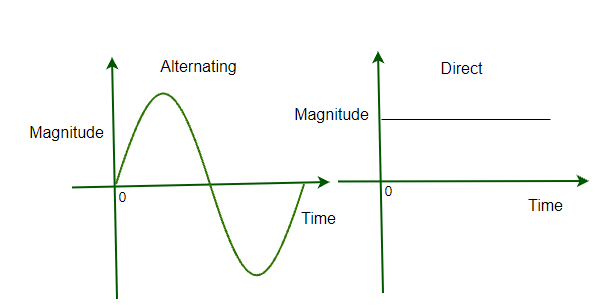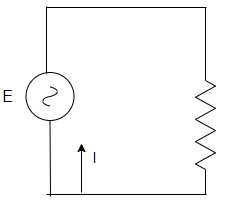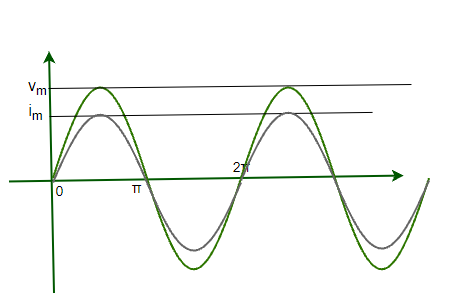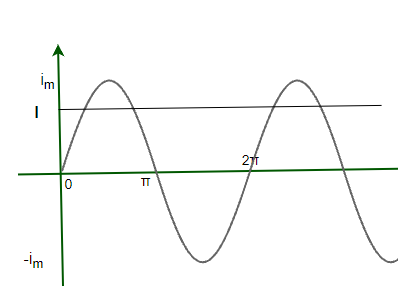GFG App
Open AppBrowser
Continue

# What is Alternating Current?

Most of the time when the topic of alternating current is discussed at this level, current and voltages do not vary with time. These currents and voltages are generated from sources that are called DC sources. In reality, in household electrical connections alternating currents are used. These currents vary with time and so does the voltage per device with them. All the appliances around us in real life are wired to work with these types of currents and voltage sources. To understand the working of these devices in real life, it becomes essential to understand the concepts behind the alternating current (AC). Let’s look at these concepts in detail.

### Alternating Current

Alternating Current is termed as a current that changes its magnitude and direction periodically. Often this periodic change of direction is defined by a sinusoidal function. The figure given below shows the graph of the magnitude of alternating current and a current from DC sources. Notice that the magnitude of the current from AC sources is changing, the negative magnitude means that the current has changed its direction, on the other hand, the graph of current from a dc source looks like a constant line.Alternating currents are also accompanied by alternating voltages. One of the advantages of using AC current over DC current is that AC current can be transmitted easily and its voltage can be increased or decreased easily by using transformers.

### Production

These currents can be generated using devices which are known as alternators. Apart from these, there are many circuits that are able to produce alternating voltages and current. One of the most basic ways to produce an AC voltage or current is by using a single coil AC generator. The generator consists of two-pole magnets and a single loop of wire which has a rectangular shape.

This setup is based on Faraday’s principle of electromagnetic induction. We are able to convert mechanical energy into electrical energy using this principle. For supplying AC, the following three wires are used:

1. Hotwire for power transmission.
2. The neutral wire is connected to the earth, this wire provides a return path for the current in hot wire.
3. The third wire is connected to the earth is linked to the metallic parts to avoid electric shock hazards.

### Alternating Current applied to a Resistor

The figure given below shows an alternating voltage source being applied to a resistor that is connected in series with the source. The source produces a sinusoidal varying potential difference across its terminals. Let us assume that this potential difference is called AC voltage. Then, this sinusoidally varying voltage can be expressed by the equation given below,

v = vmsinωt

Here, vm is the amplitude of the oscillating voltage and ω denotes its angular frequency.The amplitude of the current in the given circuit is given by,

i =The figure is given below plots both of the values on the graph. Notice that both current and voltage go to maximum and become zero at the same time. This means that they have zero phase difference.It can be inferred from the figure that the average current is also zero in a single cycle is zero.

### Power Dissipation

Even though the average current through the cycle is zero, but that does not mean that average power dissipation through the cycle is also zero. Dissipation of electrical energy is there. It’s known that Joule’s heating is given by i2R and depends on i2. This term is always positive irrespective of the sign of “i”. Thus average dissipation cannot become zero.

p = (im)2sin2ωtR

This is the instantaneous power in the circuit. Average power dissipation is given by,

pavg = 1/2(im)2R = (vm)2/2R

Expressing this expression of power similar to the usual expression.

pavg = I2R = V2/R

Whereand### Sample Problems

Question 1: A light bulb is rated at 100W for a 200V supply. Find the resistance of the bulb.

The Average power is given by,

P = V2/R

Given:

V = 200

R = ?

P = 100

Plugging these values in the equation,

P = V2/R

⇒ 100 = (200)2 /R

⇒ R = 400 Ohms

Question 2: Find the expression for the current flowing in the circuit with resistance 5 ohms. The voltage source works on the expression given, v = 10sin(30t)

For calculating the value of the current Kirchhoff’s law can be used.

∑ε(t) = 0

Applying this law to the circuit shown above,

v + iR = 0

10sin(30t) + i5 = 0

i = -2sin(30t)

Question 3: Find the expression for the current flowing in the circuit with a resistance of 20 ohms. The voltage source works on the expression given, v = 5sin(20t)

For calculating the value of the current Kirchhoff’s law can be used.

∑ε(t) = 0

Applying this law to the circuit shown above,

v + iR = 0

5sin(20t) + i(20) = 0

i = -0.25sin(30t)

Question 4: Average power dissipation in a circuit is given as 100W. The resistance of the circuit is 10 ohms. Find the peak value of the voltage in the circuit.

The Average power is given by,

P = V2/R

Given:

V = ?

R = 10

P = 100

Plugging these values in the equation,

P = V2/R

⇒ 100 = (V)2 /10

⇒ V = √1000

V = 10√10 V

This is the rms value of the voltage.

Peak Value will be,

Vpeak = Vrms√2

⇒ Vpeak = 10√20

⇒ Vpeak = 20√5 V

Question 5: Average power dissipation in a circuit is given as 200W. The resistance of the circuit is 20 ohms. Find the peak value of the voltage in the circuit.

The Average power is given by,

P = V2/R

Given:

V = ?

R = 20

P = 200

Plugging these values in the equation,

P = V2/R

⇒ 200 = (V)2 /20

⇒ V = √4000

V = 40√10 V

This is the rms value of the voltage.

Peak Value will be,

V = Vrms√2

⇒ V = 40√20

⇒ V = 80√5 V

My Personal Notes arrow_drop_up